Voltage Dividers

Voltage dividers are just particular combinations of resistors in series when connected to ideal voltage and current sources.

While the math of solving these circuits has been covered in previous sections, these combinations (or near approximations) have earned their own name because they appear often enough to be a useful shortcut when analyzing many larger circuits. We'll start with the ideal case, look at the non-ideal case, and then show when approximations are useful in design and analysis.

When you see an experienced electrical engineer take a quick glance at an analog schematic and quickly identify what's going on, they're most likely doing voltage divider approximations in their head -- sometimes without realizing it!

Ideal Voltage Divider

An ideal voltage divider is just two resistors in series. Physically, this is occasionally implemented as a potentiometer, which splits a single physical resistor in two with a physically moveable conductive center contact. Other times, it's two fixed resistors.

When a voltage is applied across the pair, the output voltage is some fraction of the input voltage: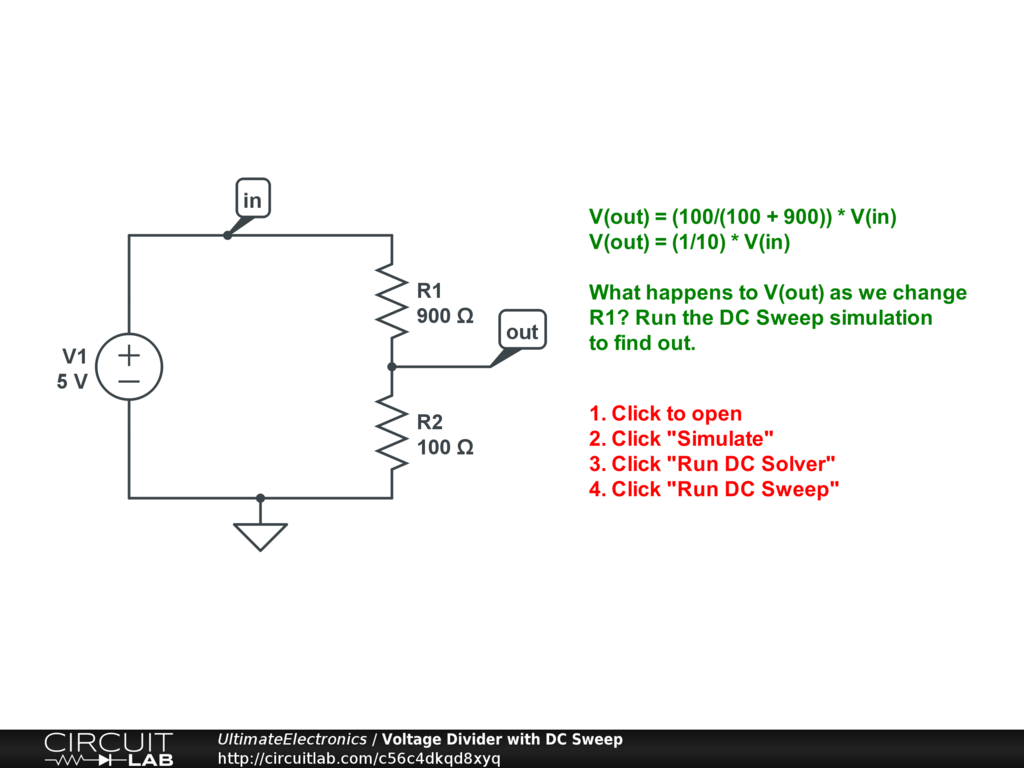Interactive Exercise Click to open and simulate the circuit above. Change the resistances and see how the output voltage changes in response.

This can be seen by using Kirchhoff's Voltage Law plus Ohm's Law to write the sum of voltage differences around the loop: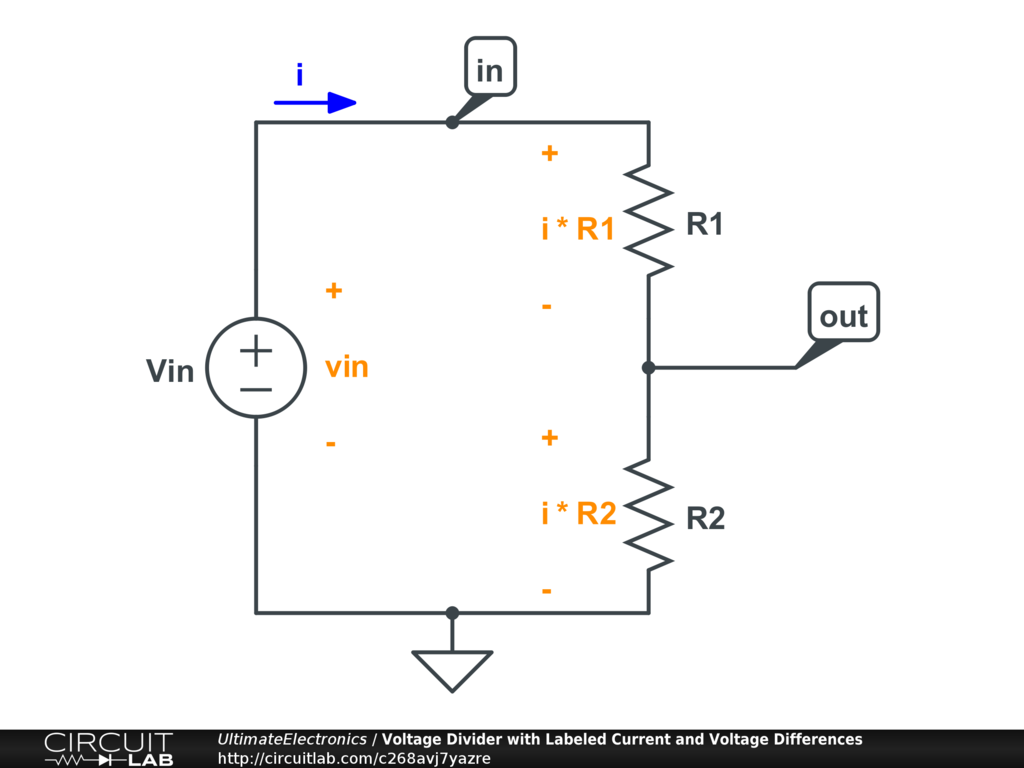\begin{align} v_{\text{in}} - i R_1 - i R_2 & = 0 \\ v_{\text{in}} & = i (R_1 + R_2) \\ \frac {v_{\text{in}}} {R_1 + R_2} & = i \end{align}

Now we can write Ohm's Law for R2, $v_{\text{out}} = i R_2$ (since the other end of R2 is grounded), and substitute in our expression for the loop current $i$ above:

$$v_{\text{out}} = v_{\text{in}} \big( \frac {R_2} {R_1 + R_2} \big)$$

For convenience, we'll pull out the fraction $f$, the voltage divider ratio:

$$f = \frac {R_2} {R_1 + R_2}$$

where $v_{\text{out}} = v_{\text{in}} \cdot f$. Since resistances can't be negative, $0 \le f \le 1$.

There are a few special cases to think about:

1. In the relatively common special case of two equal resistances $R_1 = R_2$, the ratio $f = \frac {1} {2}$.
2. If $R_1 \gg R_2$ the ratio $f \rightarrow 0$.
3. If $R_1 \ll R_2$ the ratio $f \rightarrow 1$.

These approximations are incredibly useful, and more detailed versions are developed in Algebraic Approximations.

A voltage divider always outputs a scaled-down version of the input voltage. Here's an example with a voltage divider driven by a sine wave from a function generator: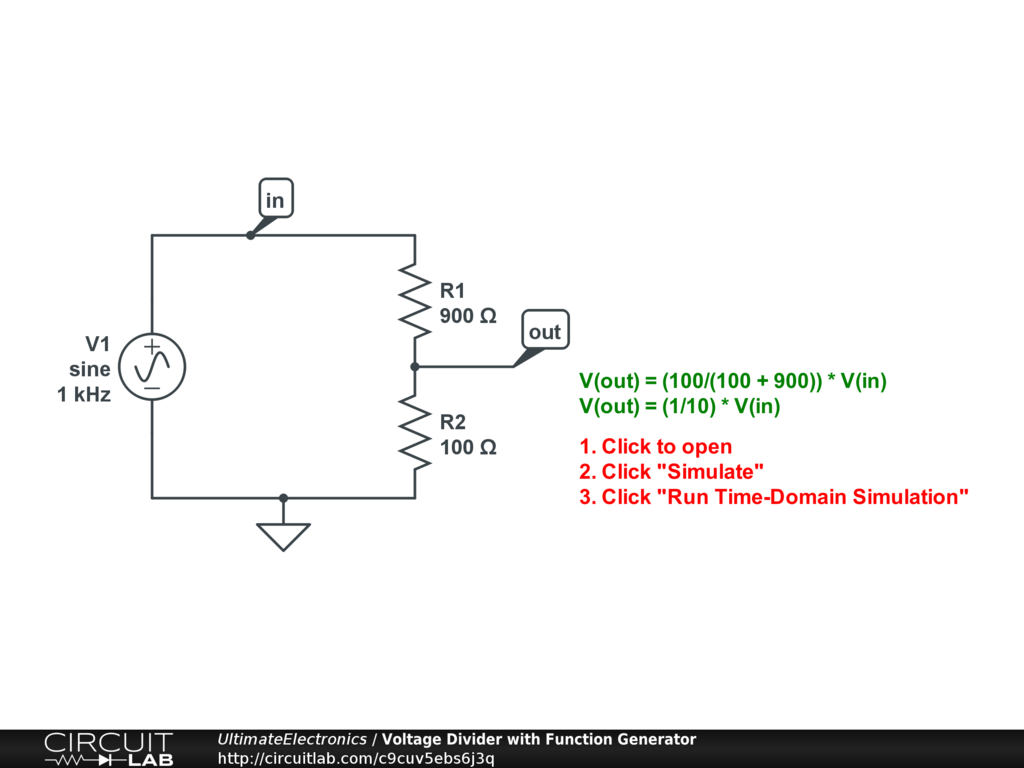Interactive Exercise Click to open and simulate the circuit above and look at the relationship between input and output signals.

Voltage Dividers on a Logarithmic Scale

Because a voltage divider outputs a multiplicatively-scaled version of the input signal, if we take the logarithm of both sides (in any base), we find that the voltage divider ratio turns into an additive constant:

\begin{align} v_{\text{out}} & = v_{\text{in}} \cdot f \\ \log(v_{\text{out}}) & = \log(v_{\text{in}}) + \log(f) \end{align}

As $f \le 1$, it will always be the case that $\log(f) \le 0$.

Here's an example with a function generator driving a voltage divider where we're plotting the voltages on a log scale: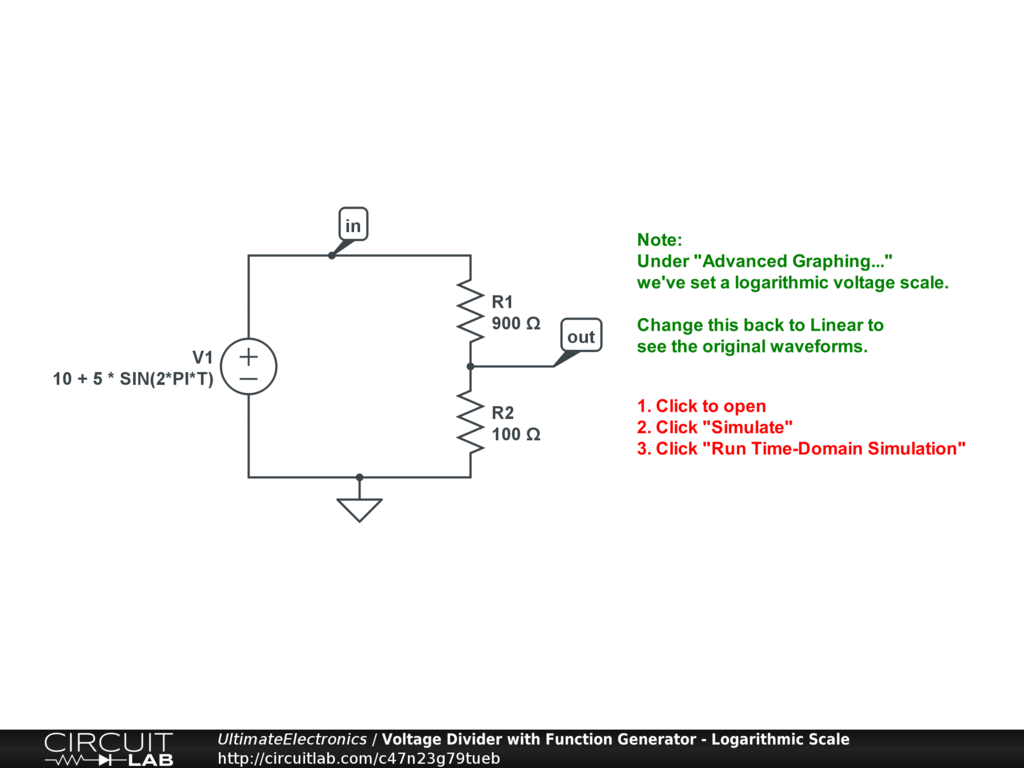Interactive Exercise Click to open and simulate the circuit above. Notice how on a log scale, the two traces appear simply vertically shifted by a constant offset. Under "Advanced Graphing," change it back to a Linear scale to see the original signal.

For this example we've taken special care to keep our input signal strictly positive, but you can also apply the same logic to the amplitude of a signal that goes both positive and negative over time. In this case, the voltage divider produces a shift in the amplitude plot in the frequency domain: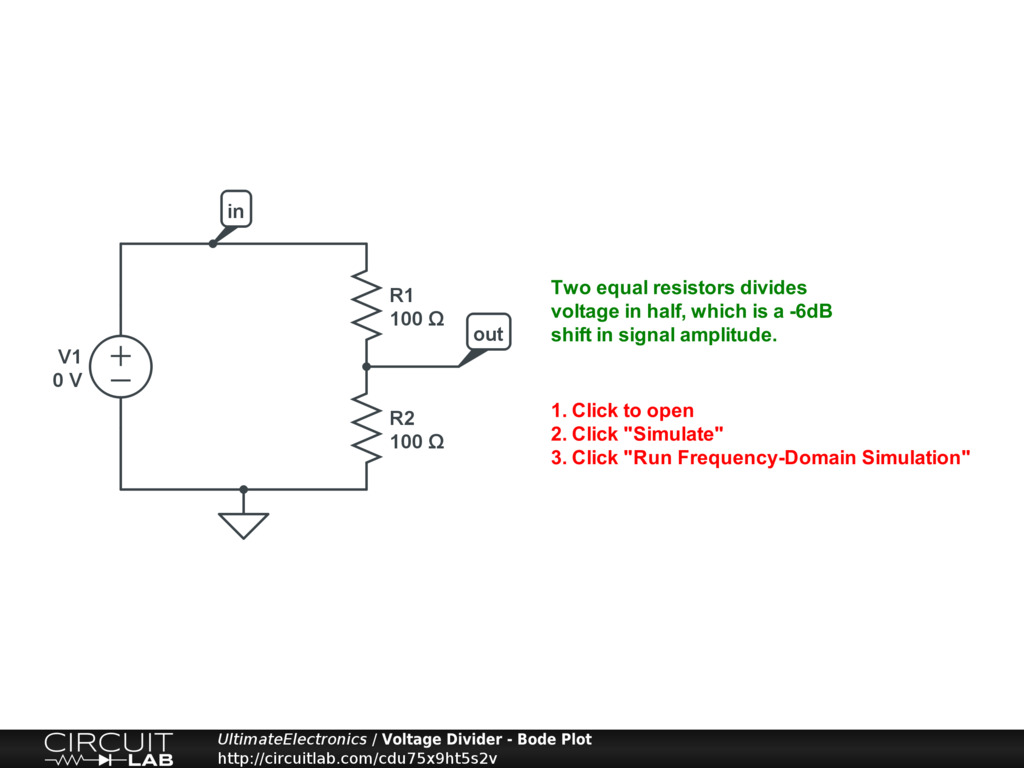Interactive Exercise Click to open and simulate the circuit above.

Running this simulation plots the input and output voltages with a $-6 \ \text{dB}$ shift between input and output amplitude. It's $-6 \ \text{dB}$ because the voltage amplitude is cut in half. See the Orders of Magnitude, Logarithmic Scales, and Decibels section for more.

Multiple-Tap Voltage Divider

Voltage dividers can be made with $N$ series resistors to have $N-1$ nodes between them. As long as there's no current entering or leaving the voltage divider chain, from the point of view of any particular tap, the resistors "above" can be combined into one, and the resistors "below" can be combined into another.

This makes it easy to create a large number of voltage ratios given a single input. For example: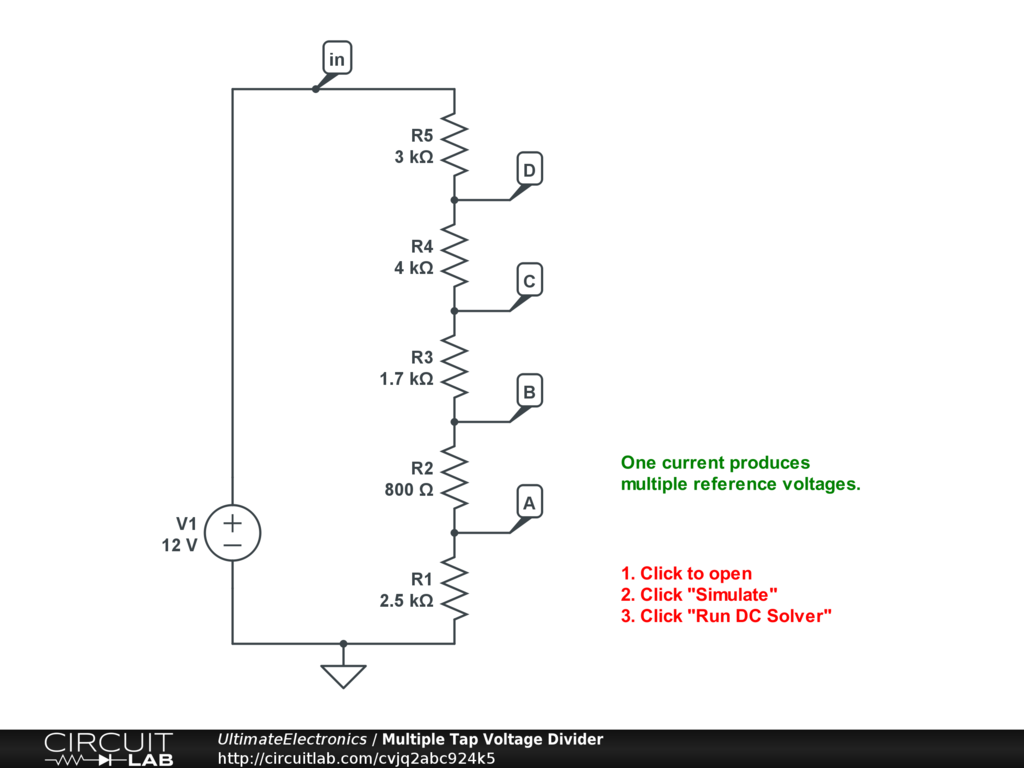Interactive Exercise Click to open and simulate the circuit above.

The schematic above uses five resistors in series. It turns a 12-volt input into four different outputs, which might be useful in a computer's power supply.

As it's a series circuit, there's only one current, and it's easily determined by considering the resistors in series:

$$i_\text{total} = \frac {v_\text{in}} {\sum R}$$

Any one resistor's voltage drop is then just:

$$\Delta v _n = R_n \cdot i_\text{total}$$

and the fraction of the total voltage drop across any one resistor $R_n$ is:

\begin{align} f_n & = \frac {\Delta v_n} {v_\text{in}} \\ f_n & = \frac {R_n \cdot i_\text{total}} {v_\text{in}} \\ f_n & = \big( \frac {R_n} {v_\text{in}} \big) \big( \frac {v_\text{in}} {\sum R} \big) \\ f_n & = \frac {R_n} {\sum R} \end{align}

The fractional voltage drop across one resistor is just the ratio of its resistance to the sum of all resistances.

For the five resistors in series above, we can calculate the voltages at each node (relative to ground) by looking at the ratio of resistance "below" that node (i.e. between that node and ground) to the total resistance of the chain. In this example with nodes and resistors as labeled above:

\begin{align} v_A & = \big( \Delta v_1 \big) && = v_\text{in} \big( f_1 \big) \\ v_B & = \big( \Delta v_1 + \Delta v_2 \big) && = v_\text{in} \big( f_1 + f_2 \big) \\ v_C & = \big( \Delta v_1 + \Delta v_2 + \Delta v_3 \big) && = v_\text{in} \big( f_1 + f_2 + f_3 \big) \\ v_D & = \big( \Delta v_1 + \Delta v_2 + \Delta v_3 + \Delta v_4 \big) && = v_\text{in} \big( f_1 + f_2 + f_3 + f_4 \big) \\ \end{align}

When we work out the solution for the circuit above:

\begin{align} v_A & = v_\text{in} \big( \frac {R_1} {R_1 + R_2 + R_3 + R_4 + R_5} \big) = 2.5 \ \text{V} \\ v_B & = v_\text{in} \big( \frac {R_1 + R_2} {R_1 + R_2 + R_3 + R_4 + R_5} \big) = 3.3 \ \text{V} \\ v_C & = v_\text{in} \big( \frac {R_1 + R_2 + R_3} {R_1 + R_2 + R_3 + R_4 + R_5} \big) = 5.0 \ \text{V} \\ v_D & = v_\text{in} \big( \frac {R_1 + R_2 + R_3 + R_4} {R_1 + R_2 + R_3 + R_4 + R_5} \big) = 9.0 \ \text{V} \\ \end{align}

Of course, for problem solving, we picked particular values that were easy to work out by hand: you may have noticed that we picked $v_\text{in} = 12 \ \text{V}$ and $\sum R = 12 \ \text{k}\Omega$ so that $i_\text{total} = 1 \ \text{mA}$.

As we'll see later, this type of circuit is not very power efficient and would not be able to supply much current. However, it would be one potentially valid way to turn a single reference voltage into a number of other proportional reference voltages, which could then be used as part of a feedback loop in an efficient switching power supply design.

Integer Ratios

One common problem solving shortcut is to notice when the resistors in a voltage divider are an integer multiple of each other.

For example, if $R_1 = 100\ \Omega, R_2 = 300\ \Omega$, then there's a 3-to-1 ratio. Whenever there's a 3-to-1 ratio, regardless of the actual values, we'll have $f = \frac {R_2} {R_1 + R_2} = \frac {3} {4}$.

This also helps for design. If you're trying to aim for a known voltage fraction $f$ which you can express as a fraction of integers, then you can quickly compute the necessary ratio of resistances.

For example, if you're trying to hit $f = 0.4 = \frac {2} {5}$, you can look for resistor values where $R_2 = 2 x, R_1 = 3 x$ for any value of $x$, so that $\frac {R_2} {R_1} = \frac {2} {3}$. (Keep reading below to learn how we pick $x$!)

In some prototyping situations, if the integers in the numberator and denominator are reasonably small, you could simply use five equal-valued resistors, putting two in series to form $R_2$ and three in series for $R_1$.

Thevenin Equivalent of the Voltage Divider

When we include a driving voltage source, we can include consider what happens when we look at the intermediate terminal of a voltage divider to find the Thevenin equivalent circuit: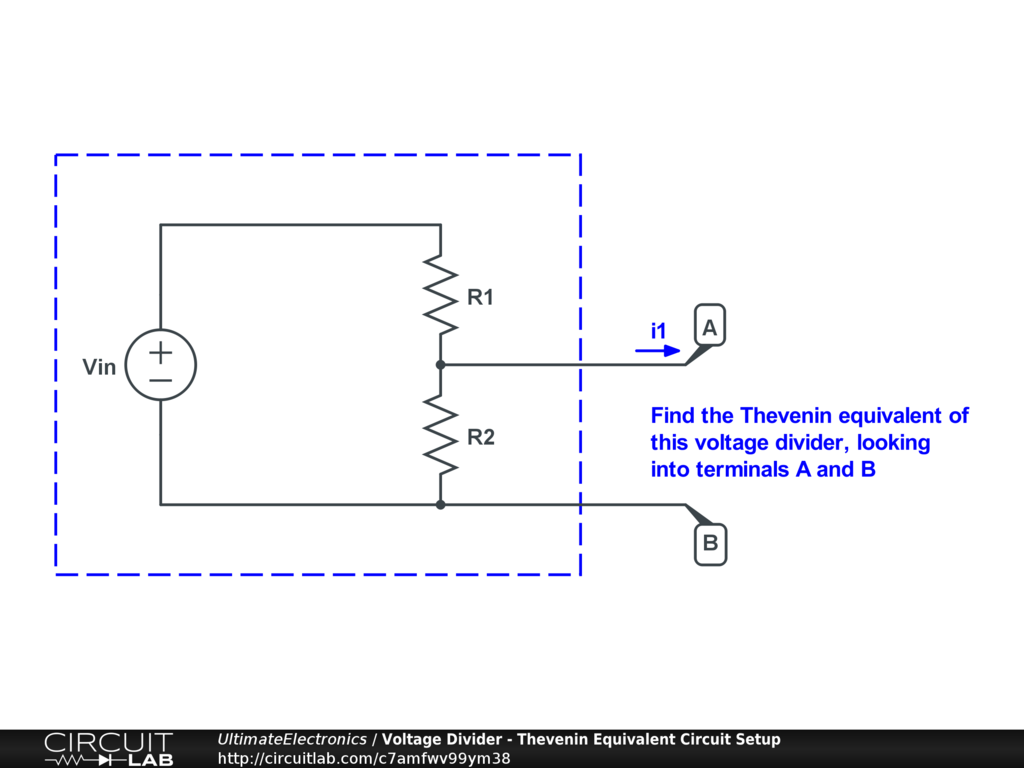The open-circuit voltage is just the voltage we'd measure across these terminals with no external current flow, which is just the fractional input voltage we calculated above:

$$V_\text{eq} = v_{\text{out,unloaded}} = v_{\text{in}} \big( \frac {R_2} {R_1 + R_2} \big)$$

Once we start to allow current to flow externally to and from these terminals, the measured voltage will change. As described in the Thevenin section, we can insert a test current source and set all independent sources to zero to calculate the equivalent resistance: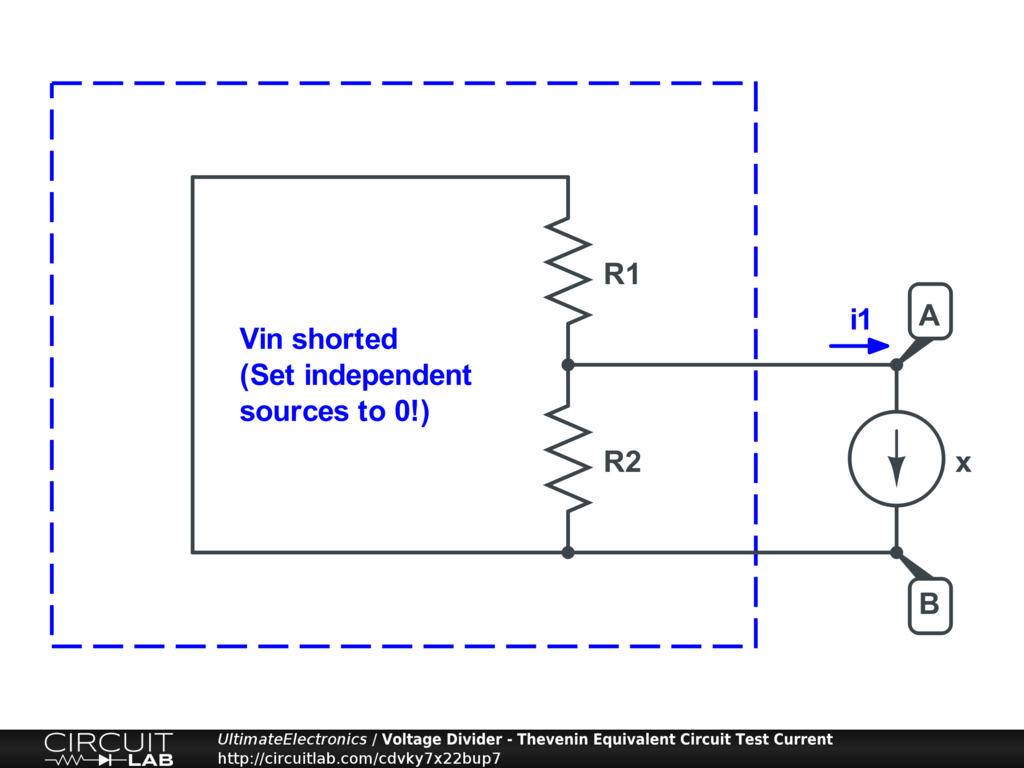From here, it's clear that the equivalent resistance is that of the two resistors in parallel:

$$R_\text{eq} = R_1 // R_2 = \frac {R_1 R_2} {R_1 + R_2}$$

Thus, the two-resistor voltage divider looks like a one-resistor Thevenin equivalent circuit: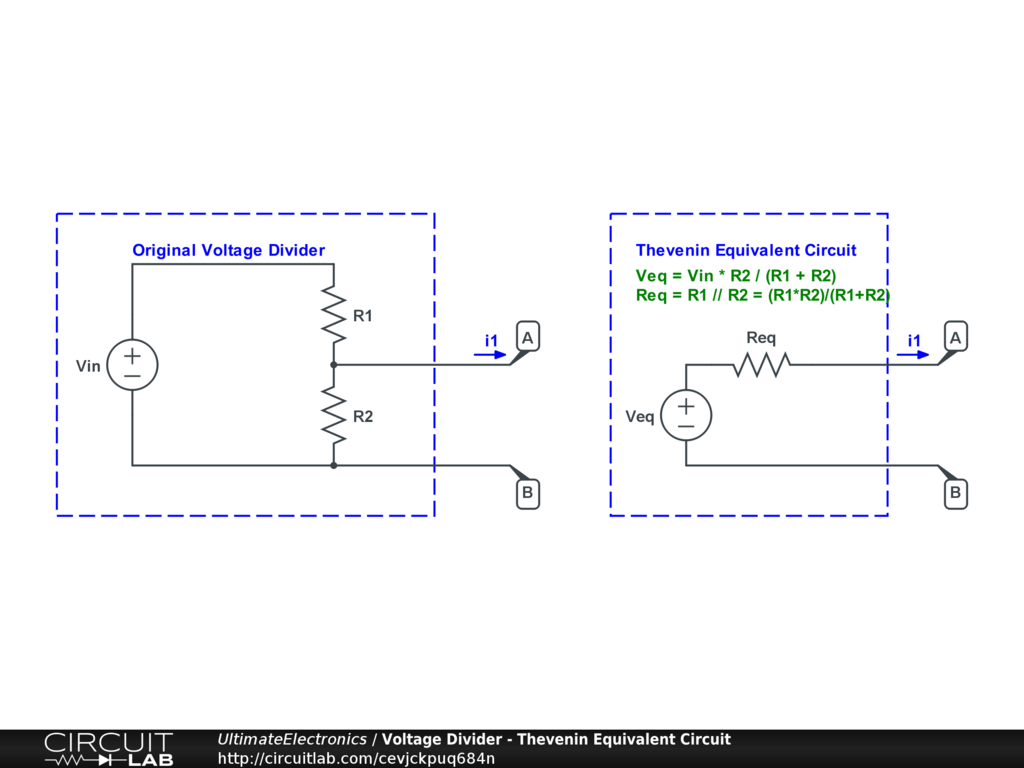Meta: Thevenin Equivalents are Useful as Voltage Dividers

We just transformed a two-resistor voltage divider into a one-resistor Thevenin equivalent circuit, which has identical current-voltage curve behavior with respect to some externally-connected load. But once we have a Thevenin equivalent circuit, often the most useful thing to do with it is to connect it to some external load. If that load is a resistor, then we've just formed a new voltage divider.

For example, we could take the three-resistor circuit on the left and consider R1 and R2 to form a voltage divider, and then replace them with their Thevenin equivalent: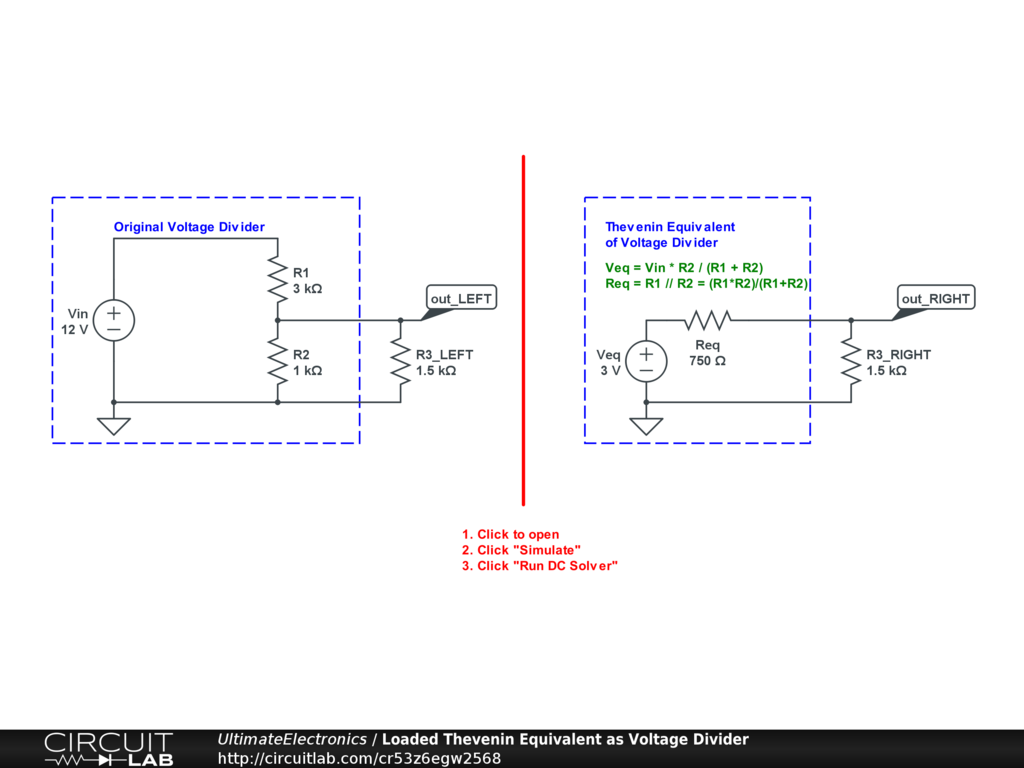Interactive Exercise Click to open and simulate the circuit above.

You should run the simulation to verify that R3 has the same current flowing through it in both cases.

Replacing a voltage divider by its Thevenin equivalent may itself form a new voltage divider, which can again be solved in the same way. This is a great problem solving technique.

The Thevenin equivalent example above is especially useful because it brings up the concept of loading: what happens when a current "load" is pulled from the middle terminal of a voltage divider?

In the Ideal Voltage Divider math above, we calculated the output voltage as a function of the input voltage $v_\text{in}$ and the voltage divider ratio $f = \frac {R_2} {R_1 + R_2}$ only. But this calculation assumed that the same current $i$ flowed through both resistors R1 and R2.

In a loaded voltage divider, this is not the case: there is some external current $i_3$ being drawn from the middle of the voltage divider: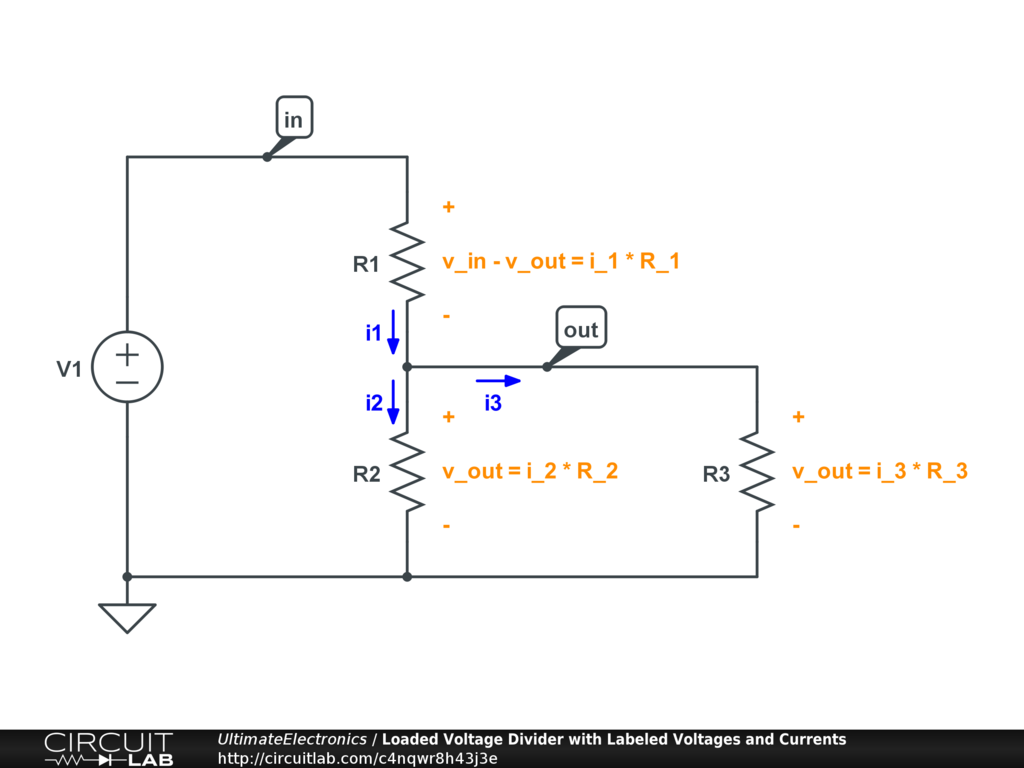As a result, if $i_3 \ne 0$, then we'll also have $i_1 \ne i_2$. This breaks the fundamental assumption we made for the ideal voltage dividers earlier.

Note that neither the circuit or the equations "know" that R3 is the external load and R2 is part of the original voltage divider. The math works out the same if we switch the names we give either. Nonetheless, thinking about it in this way is how loaded voltage dividers become useful for circuit design and analysis, as we'll see below.

Solving by Hand

Now, when we apply our rules for Solving Circuit Systems, we have different current values in different circuit branches. Our Kirchhoff's Current Law equation plus our two Ohm's Law equations form the following system of three equations:

\begin{align} i_1 & = i_2 + i_3 \\ v_{\text{in}} - v_{\text{out}} & = i_1 R_1 \\ v_{\text{out}} & = i_2 R_2 \end{align}

We'd like to solve this system for a single expression of $v_\text{out}$ as a function of $v_\text{in}, R_1, R_2, \text{and} \ i_3$. (We'll treat $i_3$ as an independent variable so we can see the effect of load current directly, rather than linking it to the voltage via the resistance $R_3$.) It's quite instructional to solve this by hand so you can see how the Solving Circuit Systems approach produces the same answer as the Thevenin approach above, so please grab a piece of paper and follow along.

First we'll re-arrange a few of the equations, taking $i_2 = i_1 - i_3$ from the first equation and substituting into the third to yield:

$$v_{\text{out}} = (i_1 - i_3) R_2$$

We'll also take the second equation and divide both sides by $R_1$:

$$i_1 = \frac {v_\text{in} - v_\text{out}} {R_1}$$

We can combine these two, substituting in for $i_1$:

$$v_{\text{out}} = (\frac {v_\text{in} - v_\text{out}} {R_1} - i_3) R_2$$

From here, we just have to do some algebra. Distribute the $R_2$:

$$v_{\text{out}} = (v_\text{in} - v_\text{out}) \big( \frac {R_2} {R_1} \big) - i_3 R_2$$

Collect the $v_\text{out}$ terms on the left-hand side:

$$v_{\text{out}} \big( 1 + \frac {R_2} {R_1} \big) = v_\text{in} \big( \frac {R_2} {R_1} \big) - i_3 R_2$$

Replace $\big( 1 + \frac {R_2} {R_1} \big) = \big( \frac {R_1 + R_2} {R_1} \big)$:

$$v_{\text{out}} \big( \frac {R_1 + R_2} {R_1} \big) = v_\text{in} \big( \frac {R_2} {R_1} \big) - i_3 R_2$$

And divide it out to isolate the $v_\text{out}$:

\begin{align} v_{\text{out}} & = v_\text{in} \big( \frac {R_2} {R_1} \big) \big( \frac {R_1} {R_1 + R_2} \big) - i_3 R_2 \big( \frac {R_1} {R_1 + R_2} \big) \\ v_{\text{out}} & = v_\text{in} \big( \frac {R_2} {R_1 + R_2} \big) - i_3 \big( \frac {R_1 R_2} {R_1 + R_2} \big) \end{align}

This equation describes the output voltage of a loaded voltage divider.

Look closely at the coefficients of $v_\text{in}$ and $i_3$:

1. The coefficient for $v_\text{in}$, $\big( \frac {R_2} {R_1 + R_2} \big)$, is just the voltage divider ratio $f$ we found earlier in the unloaded case. If $i_3 = 0$, then the divider is unloaded, and the overall equation simplifies to be the same as we found at the beginning of this section.
2. The coefficient for $i_3$ is equal to $\big( \frac {R_1 R_2} {R_1 + R_2} \big) = R_1 // R_2$, the parallel resistance of the two resistors! (Yes, parallel -- even though R1 and R2 appear to be in series in the voltage divider!) This is the same counterintuitive result we just found in the Thevenin Equivalent section above.

This example shows that being able to solve a circuit from the KCL and KVL may require a bit of algebraic legwork, but it always works and it always gives the right answer. If you're unsure whether you're applying the Thevenin equivalent process correctly, you can always insert the test current source $i_3$ and proceed to solve the circuit by hand.

Parallel Resistance Intuition

What's the intuition behind the load current in a loaded voltage divider causing a voltage drop proportional to the parallel resistance of R1 and R2, which (before considering the load) appear to be in series?

A quick way to remember this is to consider the extreme cases and work backwards.

At one extreme, consider $R_1 = 0$ or $R_2 = 0$ (but not both at once). In this case, the output voltage won't change at all with $i_3$. Zero resistance in parallel with a non-zero resistance is always zero equivalent resistance. In this extreme, the load is directly connected (with zero resistance) to one end of the voltage source.

Here's how the loaded voltage divider simplifies at the zero-resistance extreme: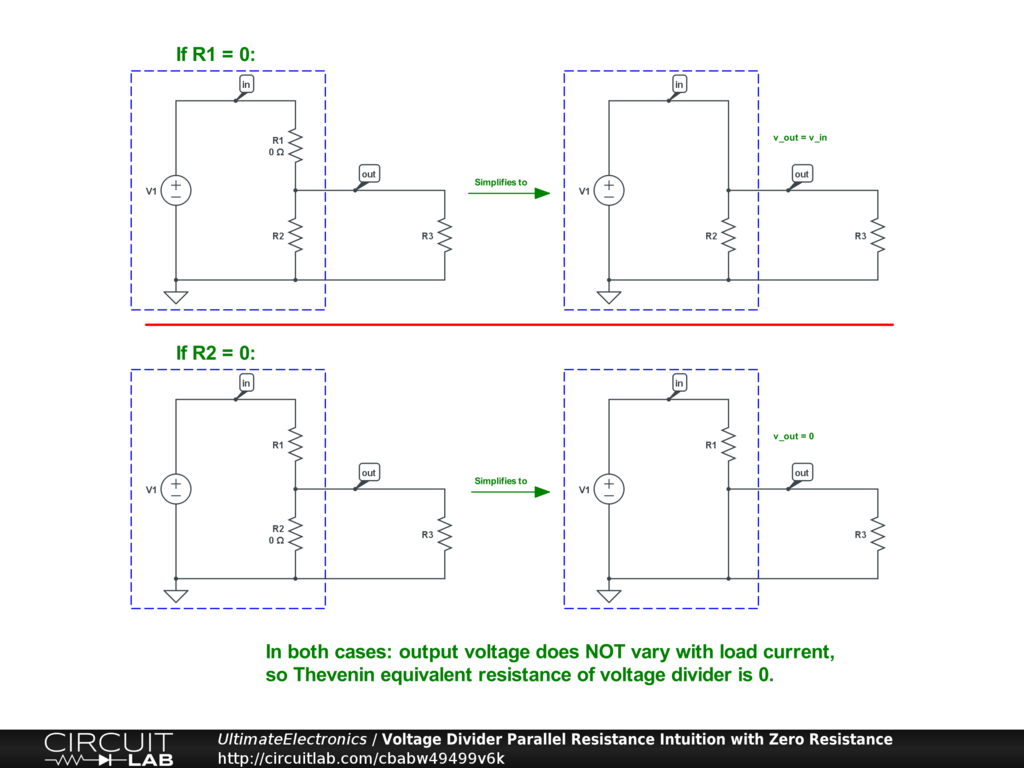At the other extreme, consider $R_1 = \infty$ or $R_2 = \infty$ (but not both at once). In this case the voltage divider is basically disconnected at one end. Infinite resistance in parallel with a finite resistance is always equal to the finite resistance.

Here's how the loaded voltage divider simplifies at the infinite-resistance extreme: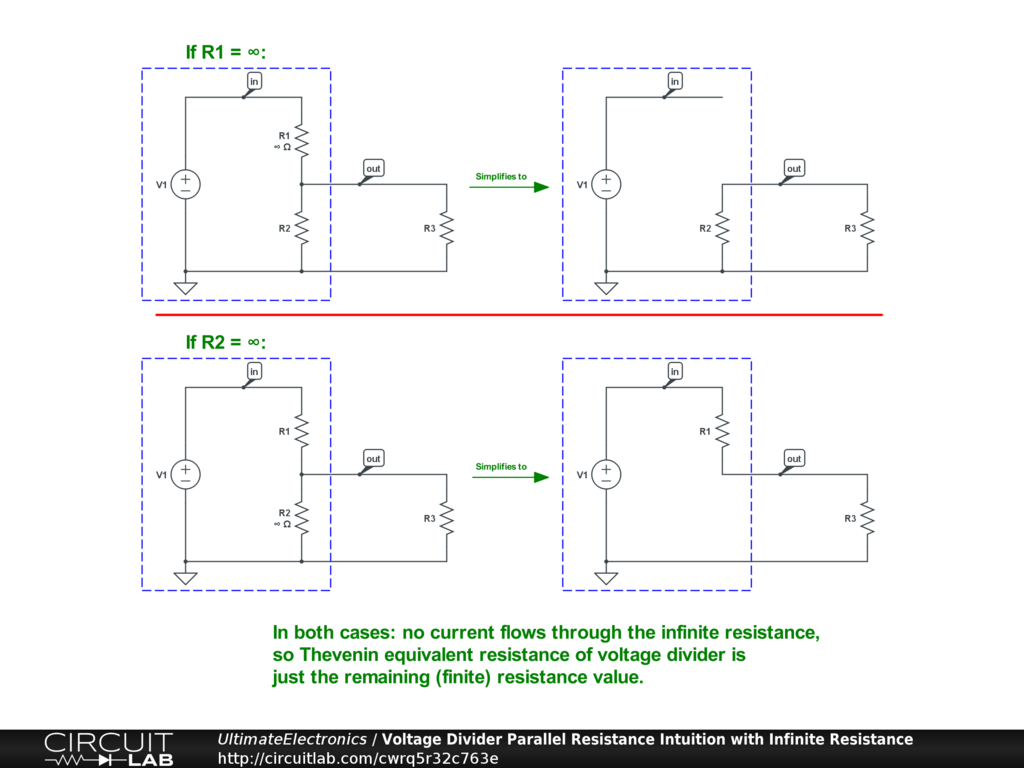The ideal voltage divider equation correctly reduces to these cases if the voltage drop due to loading is proportional to the parallel resistance $R_1 // R_2$.

What about a case in the middle, where both resistances are finite? One way to think about it is that a small increase in the load current $i_3$ has a dual effect: increasing $i_1$ and reducing $i_2$. In fact, neither of the two currents $i_1$ or $i_2$ need to change by the full magnitude of the change in $i_3$, because they literally split the difference (with opposite sign, but that's just a matter of labeling currents):

$$\Delta i_1 - \Delta i_2 = \Delta i_3$$

For example, if $R_1 = R_2$, then they'll split the difference equally, with $\Delta i_1 = \frac {1} {2} \Delta i_3$, and $\Delta i_2 = - \frac {1} {2} \Delta i_3$. Because the magnitude of the change in current through each resistor is smaller, its change in voltage is also smaller, simply due to Ohm's Law.

This illustration shows how the load current is split equally by both of the voltage divider's resistors when $R_1 = R_2$: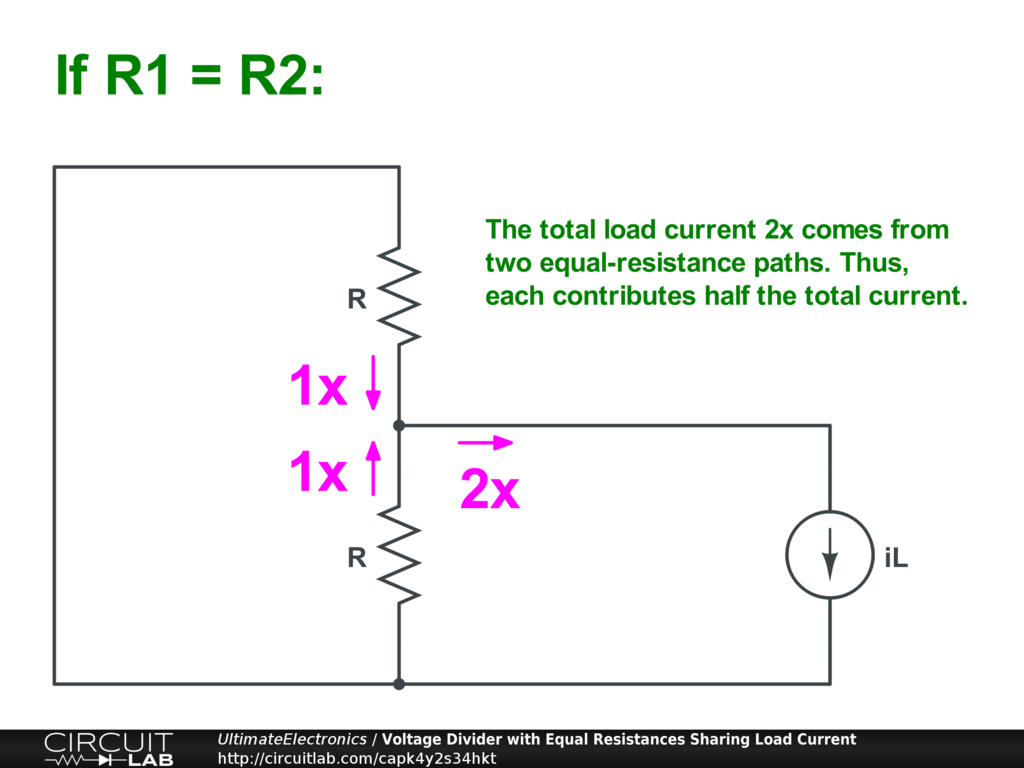We can simulate this effect numerically: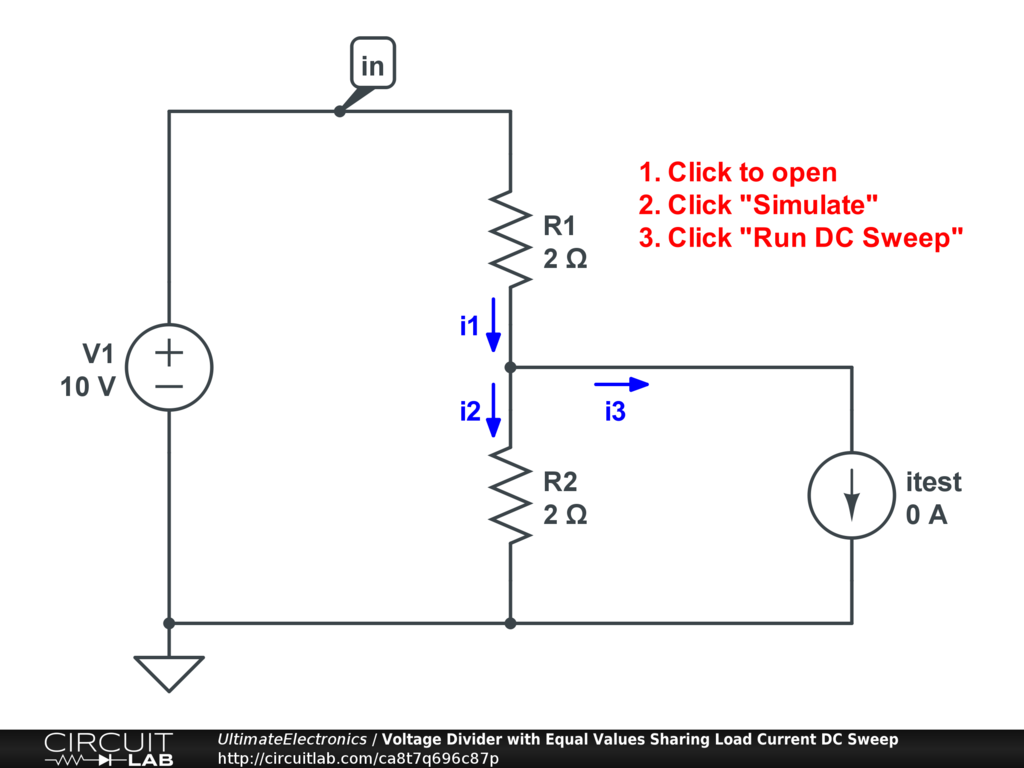Interactive Exercise Click to open and simulate the circuit above. Notice that the slope of the individual currents for R1 and R2 is half the slope as that for the test current. Change the resistances and see how the behavior changes in response.

Voltage Divider Approximations

In practice, essentially all real-world voltage dividers are loaded: something will be connected to the middle terminal so that the intermediate point is useful -- or else we would have simply used a single resistor!

However, as seen in the loaded voltage divider equation, the math for determining $v_{\text{out}}$ becomes significantly more complicated once we introduce a load current $i_3$. It'd be nice to know when it is and isn't safe to approximate a voltage divider as being approximately unloaded.

The answer comes from considering the additional voltage drop due to loading to be an approximation error $v_\text{err}$:

\begin{align} v_{\text{out}} & = v_\text{in} \big( \frac {R_2} {R_1 + R_2} \big) - i_3 \big( \frac {R_1 R_2} {R_1 + R_2} \big) \\ v_{\text{out}} & = v_\text{out,ideal} + v_\text{err} \\ \end{align}

So if we focus on only the error term:

\begin{align} v_\text{err} & = - i_3 \big( \frac {R_1 R_2} {R_1 + R_2} \big) \\ v_\text{err} & = - i_3 (R_1 // R_2) \end{align}

If we know the maximum load current magnitude $|i_{3,\text{max}}|$, and we can decide the maximum voltage error $|v_\text{err,max}|$ we're willing to tolerate, then we can put a bound on $(R_1 // R_2)$:

\begin{align} |i_{3,\text{max}}| (R_1 // R_2) & \le |v_\text{err,max}| \\ (R_1 // R_2) & \le \frac {|v_\text{err,max}|} {|i_{3,\text{max}}|} \\ \end{align}

If our tolerable voltage error is small, the resistances will also have to be small. If our tolerable load current is large, this will also force the resistances to be small. Defining this in terms of maximum tolerances means that even if the actual load current varies, as long as it's smaller than the maximum load current we designed for, we can be confident that our voltage divider will still be within our design bounds.

This is incredibly useful in practice because it means that we don't have to design our entire system at once. Approximations let us partition a large design problem into a series of smaller ones where we're free to solve one part at a time. This has two benefits:

1. Faster design time. It's often easier to solve two small subproblems than a single large one.
2. More robust performance. The solution we reach by decomposing our system into modules with error bounds may be more tolerant of unintented component variance because it was already designed with calculated error bounds in mind. In contrast, an optimized-all-at-once solution may be surprisingly fragile when some component is 5% different from its desired value. (This fragility risk is especially prevalent once we start adding any nonlinear circuit elements, such as transistors and amplifiers.)

LED Current Controller Example

Let's try a common example design problem where we have to design a voltage divider as part of a larger circuit.

We'll design a constant-current controller for an LED (Light Emitting Diode), where we want to make sure the LED draws no more than 20 mA of current to prevent it from burning out. Manufacturing variations means we don't know the exact LED voltage that will occur at, so we need current-based control of the LED, even though we have a regulated voltage as our power supply.

While saving the details for a later chapter, one way to create a practical current source is to use a BJT (Biploar Junction Transistor) with a resistor for feedback. We need to fix one terminal (the base) of this transistor at 1.7 V, and we have a 5 V power supply. We can use a voltage divider to do that: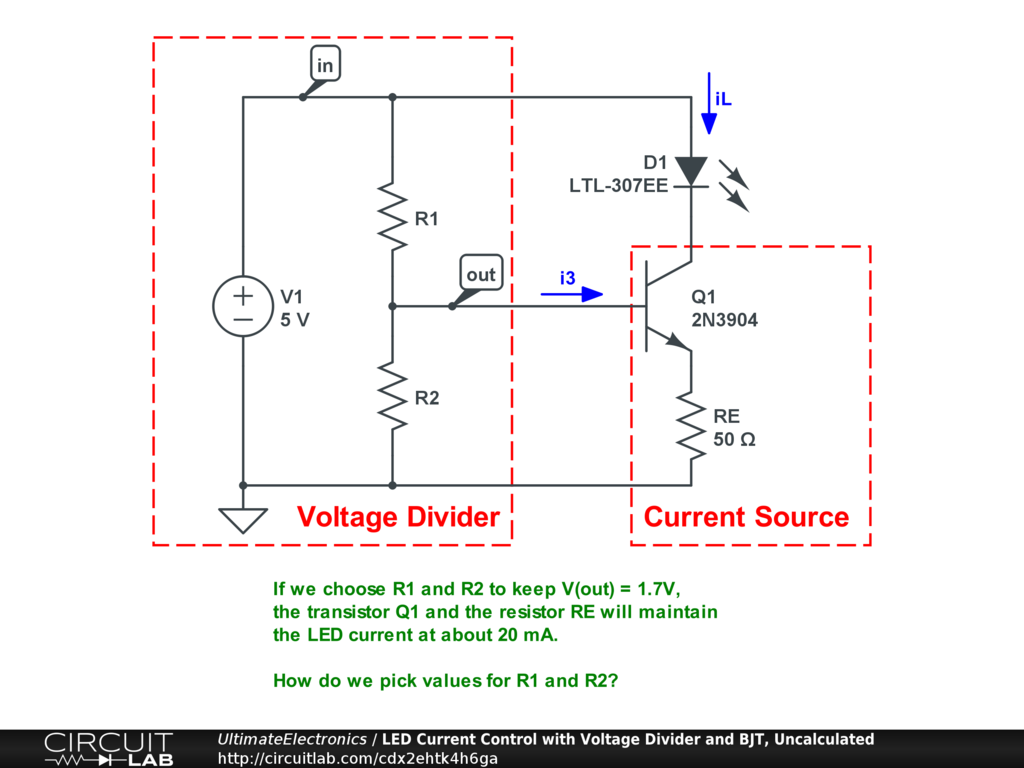We've split the circuit into basically three units: the voltage supply and voltage divider (V1, R1, and R2), the transistor-based current source (Q1 and RE), and the load we're trying to control (D1).

We now need to solve for the unknown resistors R1 and R2 to set the appropriate base bias voltage for our transistor. In reality, the voltage divider is loaded, which requires much more information to calculate the exact base voltage. Per the loaded voltage divider equation above, we'd have to know the base current too, and this would rapidly get complicated, as that circularly is dependent on the resistances R1 and R2 as well as properties of the transistor and LED themselves. The calculation complexity goes up exponentially fast!

Instead, let's make the assumption that we can treat the R1 and R2 voltage divider as approximately unloaded and solve for our desired output voltage fairly simply:

\begin{align} \frac {v_\text{out}} {v_\text{in}} = f & = \frac {R_2} {R_1 + R_2} \\ f (R_1 + R_2) & = R_2 \\ f R_1 & = R_2 (1 - f) \\ \frac {R_2} {R_1} & = \frac {f} {1 - f} \\ \frac {R_2} {R_1} & = \frac {\frac {v_\text{out}} {v_\text{in}}} {1 - \frac {v_\text{out}} {v_\text{in}}} \\ \frac {R_2} {R_1} & = \frac {v_\text{out}} {v_\text{in} - v_\text{out}} \end{align}

so if we know $v_\text{in} = 5 \ \text{V}$ and $v_\text{out} = 1.7 \ \text{V}$, then:

\begin{align} \frac {R_2} {R_1} & = \frac {1.7} {5 - 1.7} \\ \frac {R_2} {R_1} & = 0.52 \end{align}

By making the assumption that we can solve the voltage divider as unloaded, we've quickly solved for the ratio $\frac {R_2} {R_1}$.

This tells us the relative resistances of the two resistors in the voltage divider in order to get the voltage we want. However, we haven't yet established their absolute values: we could pick $\big( R_1 = 10\ \Omega, R_2 = 5.2\ \Omega \big)$ or $\big( R_1 = 1\ \text{M}\Omega, R_2 = 520 \text{k}\Omega \big)$ and both would satisfy our ratio requirement.

Choosing where to fix these absolute values has practical engineering tradeoffs at both extremes:

1. If the resistances are too small: the voltage divider itself will consume a large amount of current ($i = \frac {5 \ \text{V}} {R_1 + R_2}$) and power ($P = \frac {(5 \ \text{V})^2} {R_1 + R_2}$). This is wasteful: it increases our power supply requirements or drains our battery faster, and we have to start thinking about resistor heat dissipation and temperature coefficients -- as well as users getting physically burned by touching these hot resistors!
2. If the resistances are too large: then the voltage error due to loading $|v_\text{err}| = i_3 (R_1 // R_2)$ will be unacceptably large, and we won't get the voltage setpoint we expect.

How do we choose?

We have an engineering tradeoff between power consumption and accuracy. The correct design approach would be to put some reasonable bound on our desired accuracy level and make sure that gives us a reasonable result in power consumption.

Let's say that we'd like our desired voltage to be within about 5% of our design target. This may seem arbitrary. A reasonable way to choose an error budget is to consider values on various orders of magnitude on either side: 50% is obviously too much error in just about all circumstances; 10% would probably be fine if we really didn't care about precision; 1% or 0.5% would require us to be a lot more precise about other components in our system, like the LED and transistor themselves, not to mention resistors which are typically specified to 1% or 5% precision. 5% is nicely in the middle!

Choosing 5% as our maximum tolerated voltage error lets us say:

\begin{align} v_\text{out} & = 1.7 \ \text{V} \pm 5\% \\ v_\text{out} & = 1.7 \ \text{V} \pm 85 \ \text{mV} \\ |v_\text{err}| & \le 85 \ \text{mV} \end{align}

From above, we know that for a loaded voltage divider, $|v_\text{err}| = i_3 (R_1 // R_2)$. We haven't covered Bipolar Junction Transistors like Q1 yet, but for now, just assume that the base current is a small fraction of the load (collector) current, probably between $100 \le \frac {i_L} {i_3} \le 200$ -- i.e. the base current is probably going to be about 0.5% to 1% of our LED current.

This very rough assumption, plus the knowledge that our desired LED load current is $i_L = 20\ \text{mA}$, combine to let us put a maximum on $i_3$:

\begin{align} i_3 & \le \frac {i_L} {100} \\ i_3 & \le \frac {20 \ \text{mA}} {100} \\ i_3 & \le 200 \ \mu\text{A} \end{align}

This puts a maximum bound on the resistance values:

\begin{align} i_3 (R_1 // R_2) & \le 85 \ \text{mV} \\ (R_1 // R_2) & \le \frac {85 \ \text{mV}} {200 \ \mu\text{A}} \\ (R_1 // R_2) & \le 425 \ \Omega \end{align}

We now know that we want the parallel resistance of R1 and R2 to be no greater than $425 \ \Omega$.

We'll again use another approximation (note: adding more error, outside our earlier bounds!) and remember that if two resistors have very different values, we can approximate their parallel resistance as roughly just the smaller resistance. Our ratio $\frac {R_2} {R_1} = 0.52$ isn't quite big enough for this to be true, but it's good enough for a "back of the envelope" solution, and lets us quickly pick some common resistor values knowing that we're looking for a roughly two-to-one ratio where the smaller is roughly in the $400 \ \Omega$ ballpark:

\begin{align} R_1 & = 910\ \Omega \\ R_2 & = 470\ \Omega \end{align}

These are cheap and common resistor values, and we get:

\begin{align} (R_1 // R_2) & = \frac {R_1 R_2} {R_1 + R_2} = 310 \ \Omega \\ \frac {R_2} {R_1} & = 0.516 \end{align}

That fulfills our voltage error constraint, and is almost exactly our desired ratio. That's close enough that other tolerances (like the individual resistors and transistor) will dominate the overall error.

Here's our final LED current control circuit with R1 and R2 in place: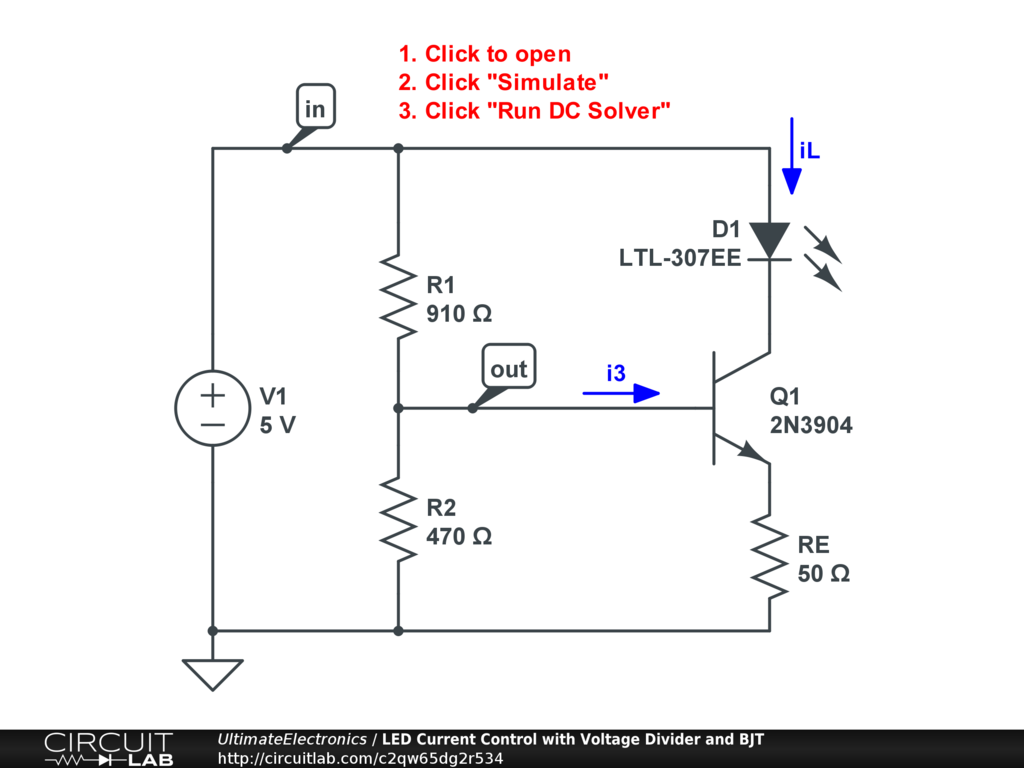Interactive Exercise Click to open and simulate the circuit above. Run the DC simulation and see how the LED current is controlled to be about 20 mA. You'll also see the calculated power dissipation in the voltage divider resistors $\text{P(R1)} + \text{P(R2)}$.

How sensitive is this circuit to the particular R1 and R2 values we selected? What if we keep the ratio $f = \frac {R_2} {R_1} = 0.52$, but choose different (both larger or both smaller) resistor values? The simulator's DC Sweep mode lets us answer this question quickly. First we define a free parameter variable $x$, and then allow the two resistances to scale with it:

\begin{align} R_1 & = x \\ R_2 & = 0.52 x \end{align}

Here's a simulation showing the LED current with various values of $x$: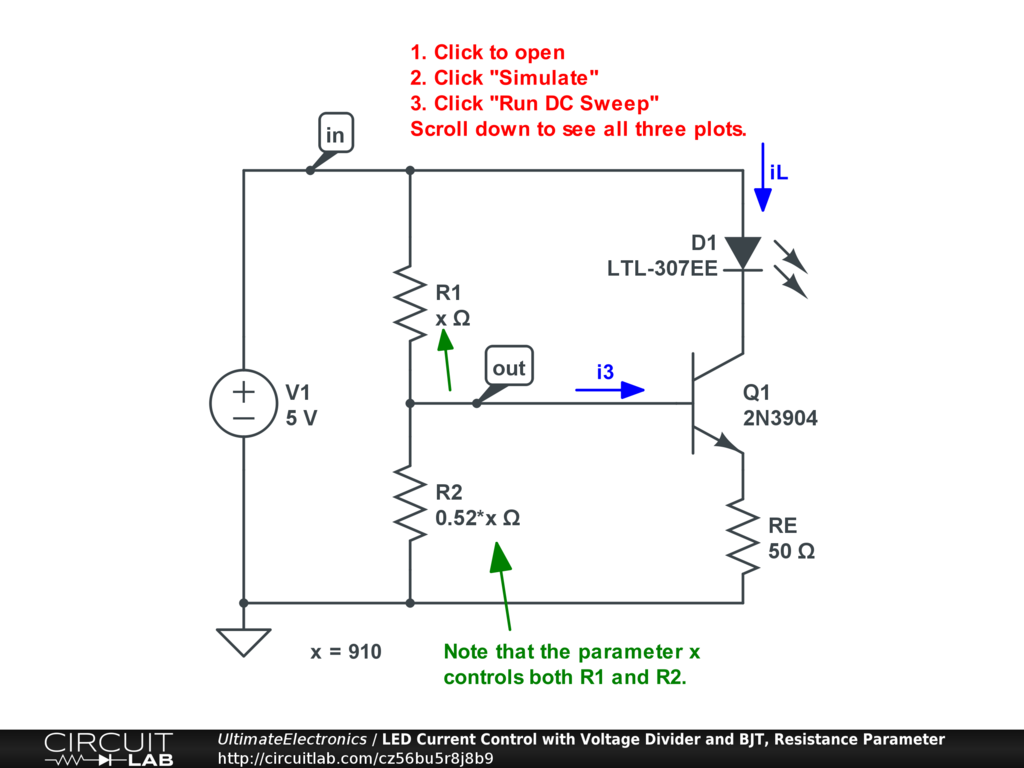Interactive Exercise Click to open and simulate the circuit above. Run the DC Sweep over the parameter $x$ and see how the LED current changes as we adjust the resistances over many orders of magnitude.

Note how the point we chose above, $x = 910$, is right before a "knee" -- visually, an almost piecewise linear change in behavior on the curve -- where performance begins to detiorate rapidly. We've plotted using a logarithmic scale for the parameter so you can see the effect of substituting resistances over many orders of magnitude. Scroll down to see the plot of the resistor heat dissipation as well, as this is something we'd generally like to minimize. Circuit simulators let you quickly see this "knee" effect, rather than calculating it at a bunch of points.

By choosing a point just a bit to the stable side of the knee, we get the consistent behavior we want, while minimizing power consumption.

Bonus: Simulating Component Tolerance

We can use the simulator to look at whether or circuit does the right thing if different component valules vary. We might expect variance in the LED, in the transistor, and in all of the resistor values. We might also expect some variance in the power supply voltage.

In each case, we can use the DC Sweep simulation mode to vary a component.

Here's what happens when the transistor is stronger or weaker: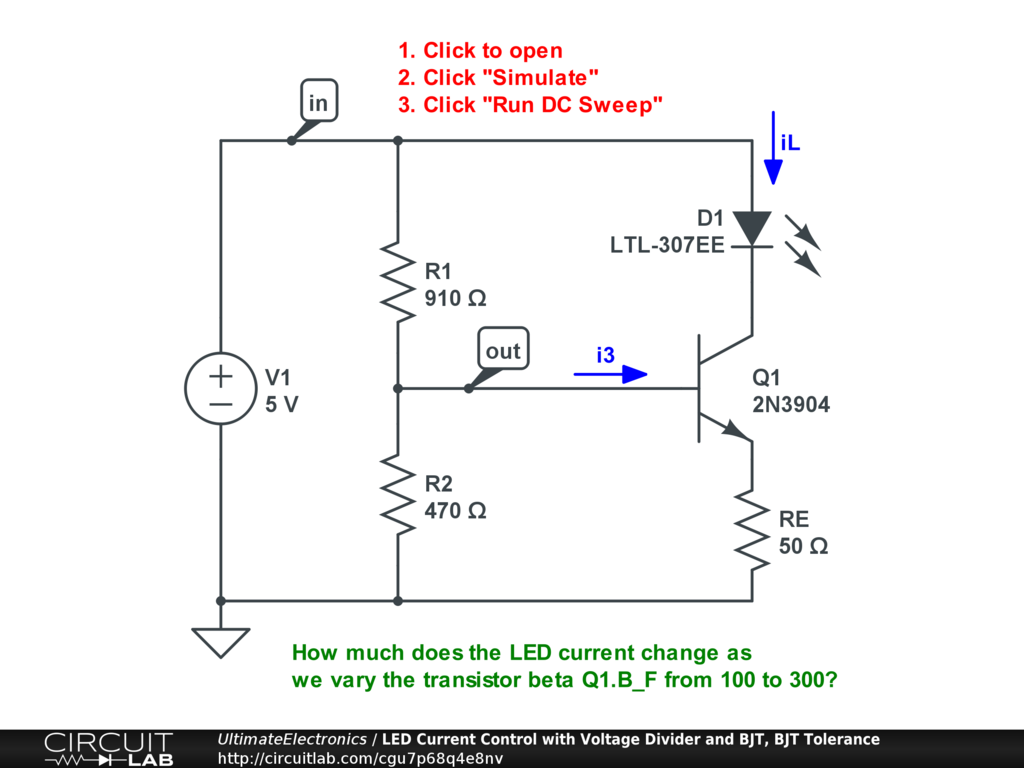Interactive Exercise Click to open and simulate the circuit above. The LED current doesn't vary much with the transistor's performance.

And here's what happens when the LED is a bit different: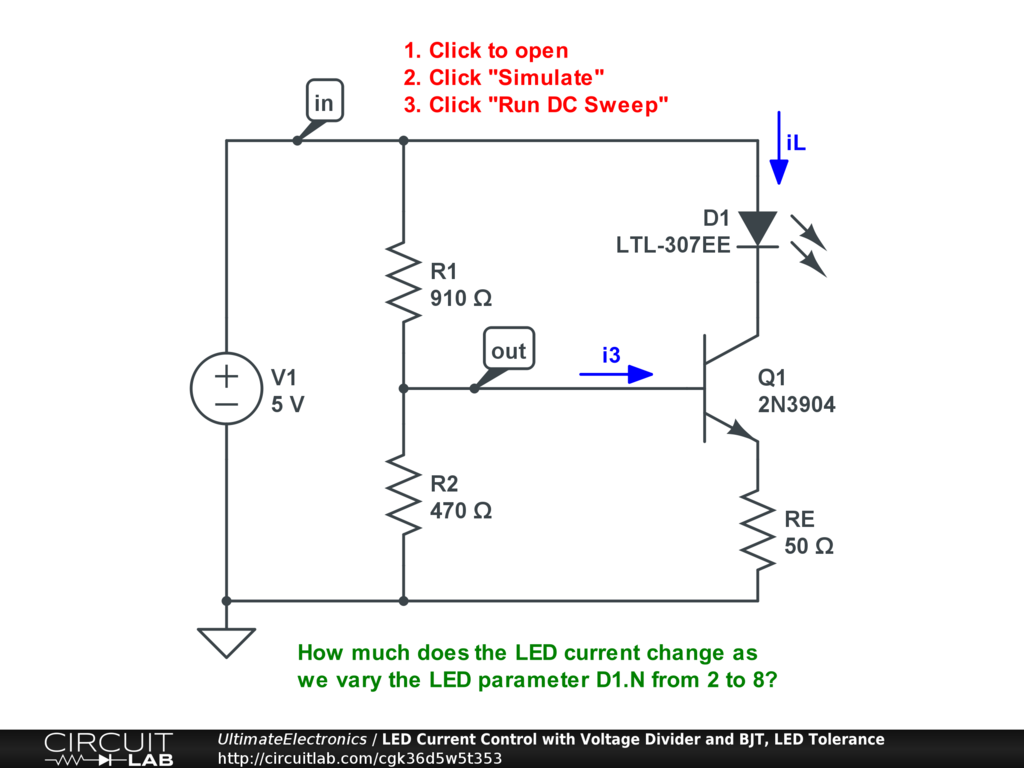Interactive Exercise Click to open and simulate the circuit above. The LED current doesn't vary much with the LED parameters either -- we've designed a good current controller!

We can also use the DC Sweep to vary two different parameters. For example, let's say that both R1 and R2 have 5% tolerance bands: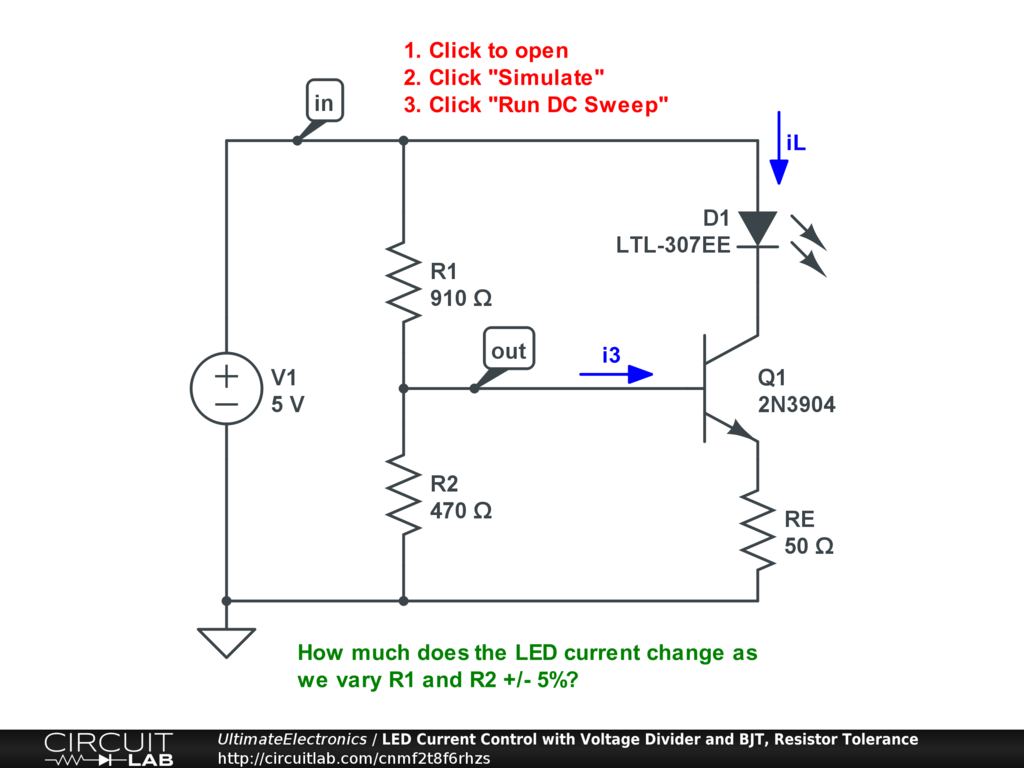Interactive Exercise Click to open and simulate the circuit above. The LED current is plotted for various combinations across R1 and R2's values.

In this case, we see an approximately +/- 10% total range of LED currents, corresponding to the combined errors of our two individual +/- 5% tolerances.

In production, we'd want to check all of our process corners -- all of our tolerance extremes across all of our components -- any make sure that the circuit still performs well enough. If it doesn't, we have to redesign or spec the circuit using tighter-tolerance components, which can increase the cost.

If you have $N$ component parameters with tolerance bands, that can theoretically be $2^N$ corners to check! In practice, we use our engineering intuition to search over the most potentially troublesome ones, and we design our circuits to be robust by design, like above.

Solving for an Unknown Resistance

One common use case for a voltage divider is for measuring an unknown resistance value. For example, we can use a fixed resistance value R1 and an unknown value R2 that we'd like to determine. (R2 might be a temperature-dependent or light-dependent resistor, for example.)

In this case, we can measure the voltage divider's output voltage $v_\text{out}$ (using a multimeter or a analog-to-digital converter, for example), and express that as a fraction of the known applied voltage $v_\text{in}$. Then we'd solve the ideal voltage divider equation for the unknown resistance as follows:

\begin{align} \frac {v_\text{out}} {v_\text{in}} = f & = \frac {R_2} {R_1 + R_2} \\ f R_1 + f R_2 & = R_2 \\ f R_1 & = (1 - f) R_2 \\ R_2 & = R_1 \big( \frac {f} {1 - f} \big) \end{align}

If we know $R_1$, and we can measure $f$, then calculating $R_2$ is straightforward.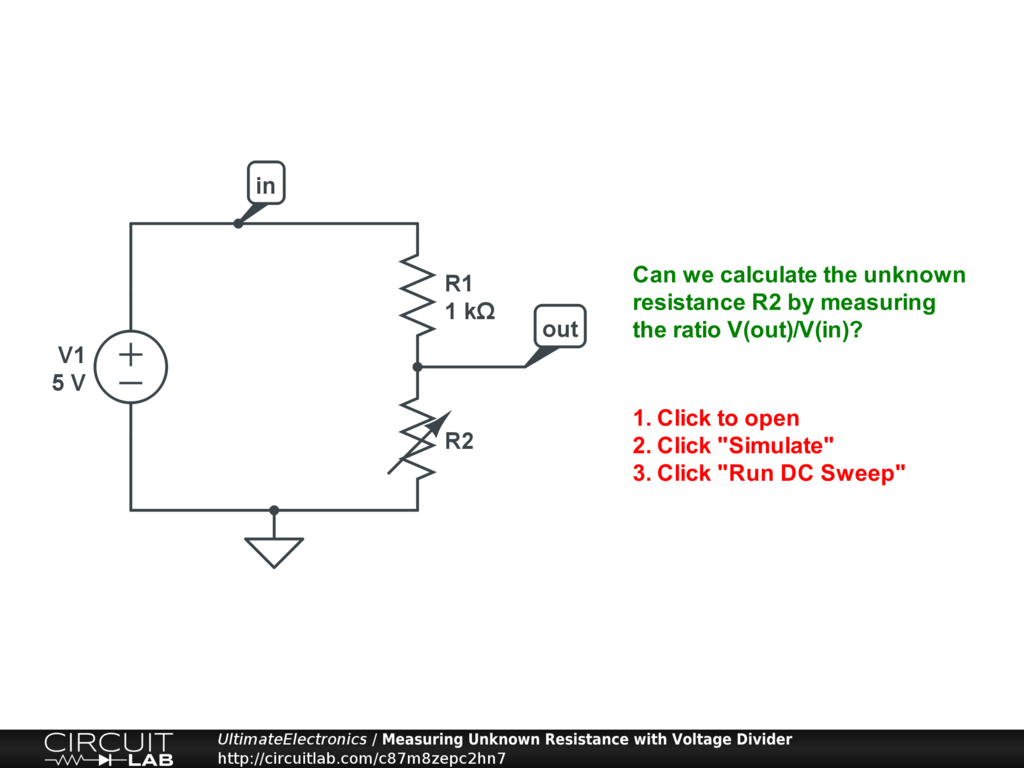Interactive Exercise Click to open and simulate the circuit above. The DC Sweep varies R2. The plot is set to show the ratio $f$ in the top plot, and then uses the equation above to back-calculate the value of $R_2$ from the measured voltages.